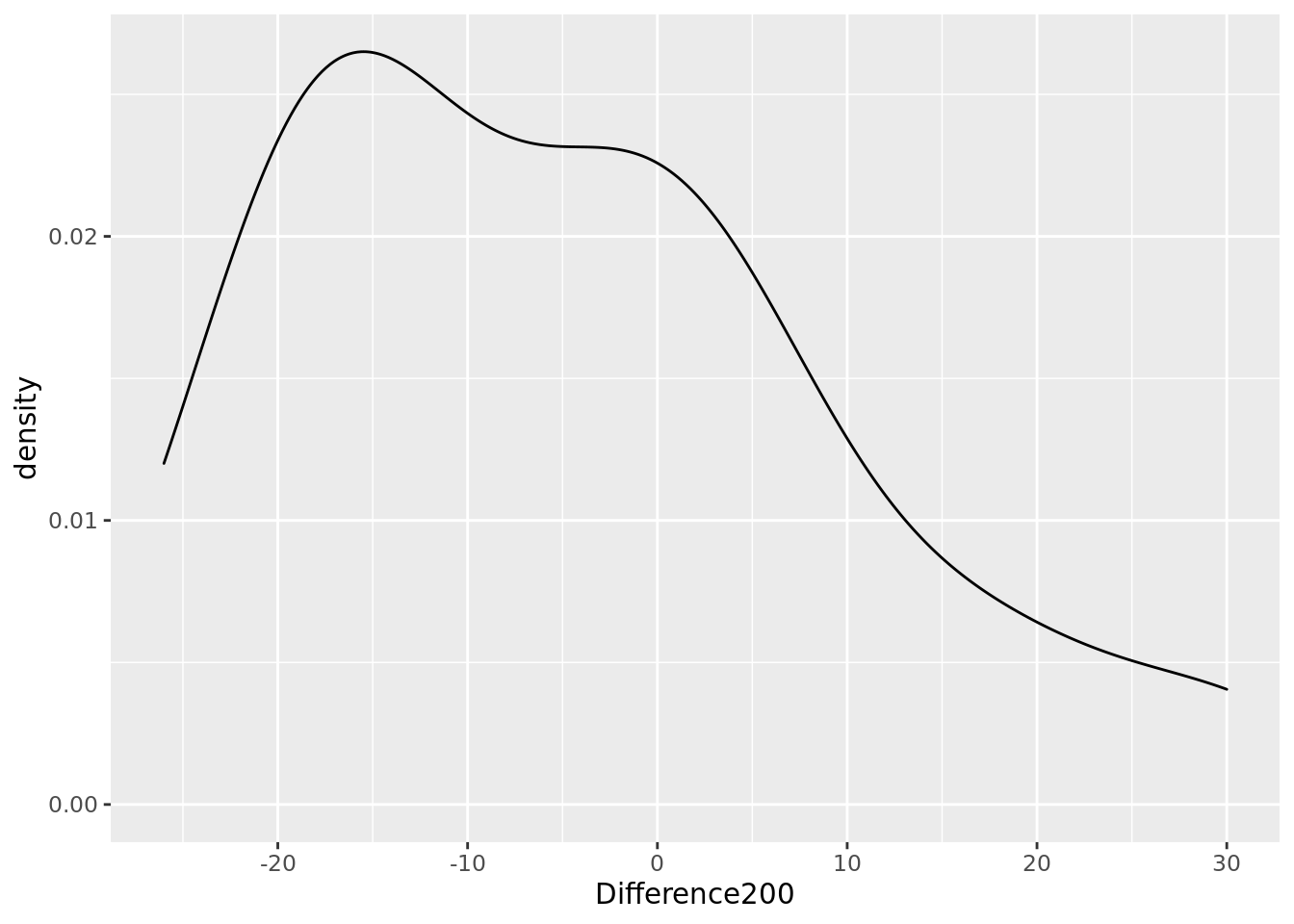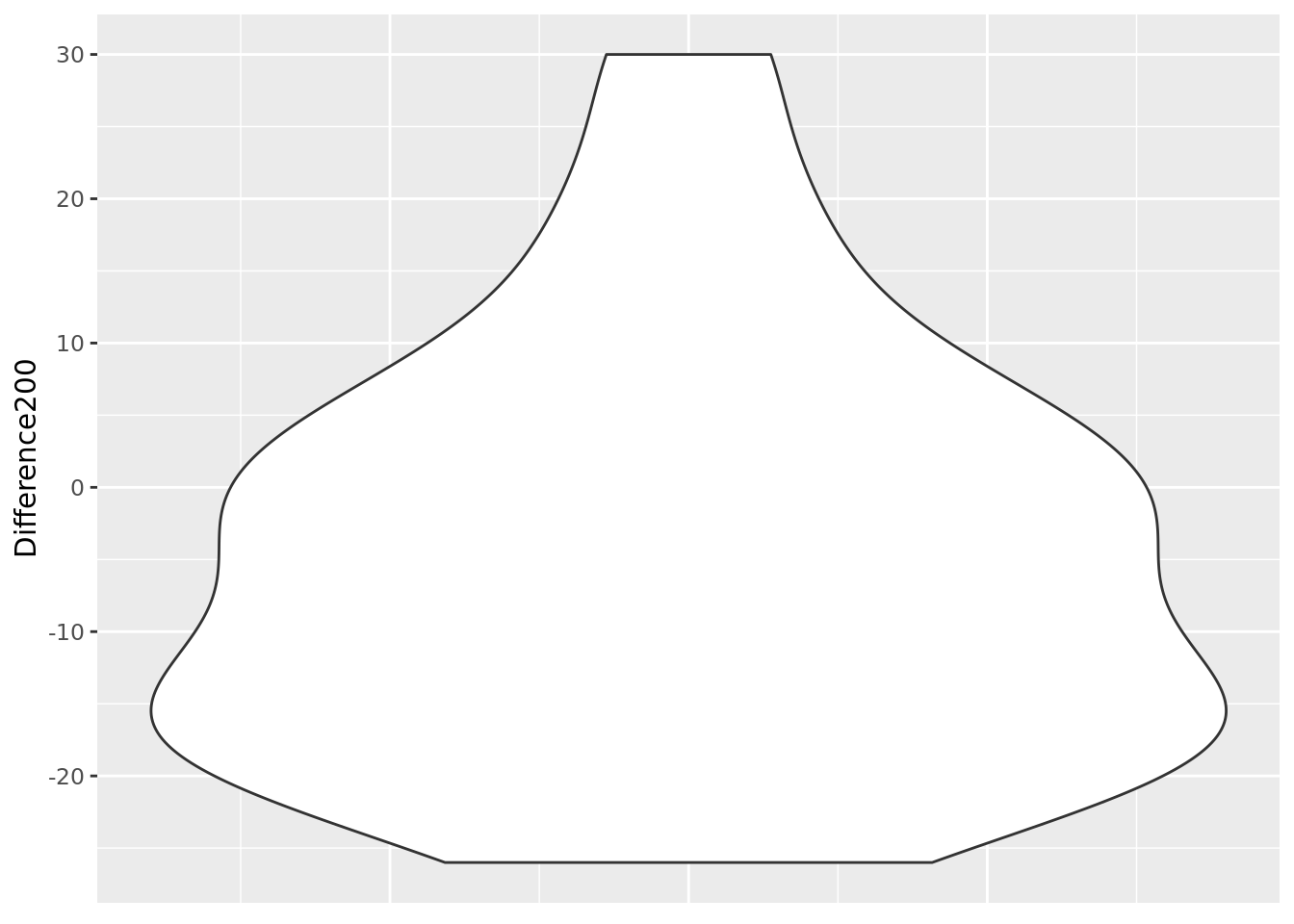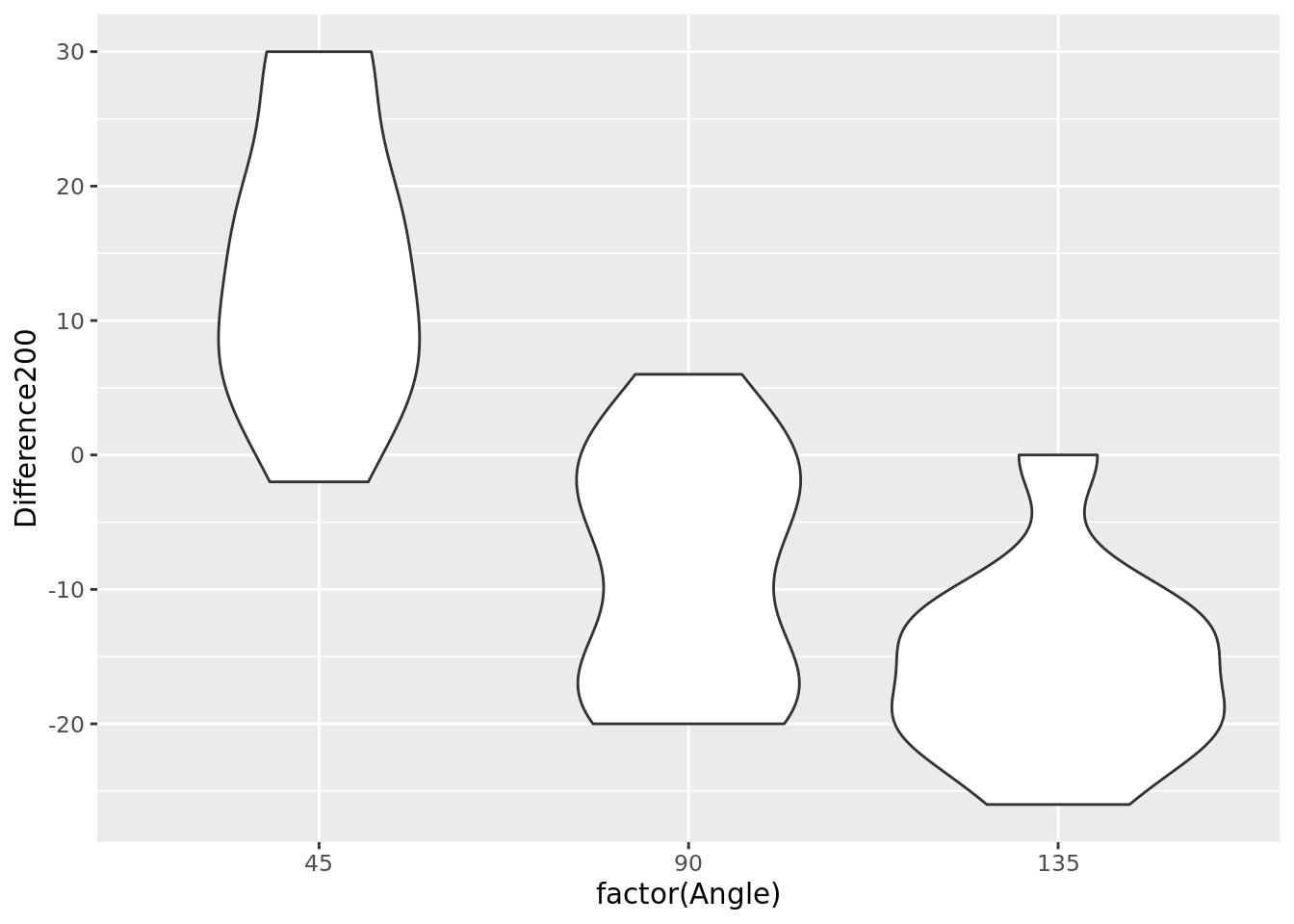## Before you start…

Before starting this exercise, you should have completed all the relevant Absolute Beginers’, Part 1 workshop exercises. If not, take a look at those exercises before continuing. Each section below also indicates which of the earlier worksheets are relevant.

## Getting the data into R

Relevant worksheet: Intro to RStudio, Entering data by hand

In order to complete this worksheet, you’ll need to have downloaded your CSV file from the PsycEL exercise. See the instructions on PsycEL for how to do this.

Plymouth University students: Create/open your project named psyc413; within that create a script file called illusions.R. Enter all commands into that script and run them from there.

Relevant worksheet: Exploring incomes

library(tidyverse)
ill <- read_csv("GG.csv")

Note: Everyone’s CSV file has a different name. For example, yours might be called 10435678 GG.csv. In the example below, you’ll need to replace GG.csv with the name of your personal CSV file.

### Inspect

Look at the data by clicking on it in the Environment tab in RStudio. Each row is one trial; here’s what each of the columns contain:

Column Description Values
Illusion The illusion presented on this trial “M” = Muller-Lyer illusion, “T” = T illusion
Angle Angle of line For Muller-Lyer, angle in degrees of small lines on left hand side of the Muller-Lyer stimulus, clockwise from 9 o’clock: 45, 90, 135. For T illusion, angle in degrees of lefthand line, clockwise from 9 o’clock: 0, 45, 90
FinalLength Final length of the line you adjusted (arbitrary units) a number; 200 if you accurately matched the other line
Difference200 FinalLength - 200 a number; 0 if you accurately matched, negative if your line was too short, positive if your line was too long

There may be an extra column called “Pattern”, but you can just ignore it. You completed 60 trials in total. Each angle was presented 10 times.

## Muller-Lyer illusion

Relevant worksheet: Exploring data, Group differences.

### Filter

To look at the Muller-Lyer illusion, you first need to filter the data, so that you just have the trials on which you did the Muller-Lyer illusion. We use the filter command to do this, as covered in the Group Differences worksheet:

mull <- ill %>% filter(Illusion == "M")

Your Muller-Lyer data are now in the data frame mull.

### Size of illusion, by angle

Did you experience the Muller-Lyer illusion? If so, did the angle of the lines affect the size of the illusion?

To look at this, you calculate the average(mean) size of your error in estimating the length of the line. You do this seperately for each angle. If you did not experience the illusion, your average error would be around zero for all angles.

The error of your judgement on each trial is in the Difference200 column of your mull data frame. The angle is in the Angle column. You can therefore calculate your mean error for each angle using the group_by, summarize and mean commands, as we covered in the Group Differences worksheet:

mull %>% group_by(Angle) %>% summarise(mean(Difference200))
# A tibble: 3 x 2
Angle mean(Difference200)
<dbl>                 <dbl>
1    45                  14
2    90                  -8.5
3   135                 -15.6

As before, you can safely ignore the “ungrouping” message that you receive.

In this example, the participant under-estimated line length with the “arrow heads” (Angle > 90), and over-estimated line length with the “arrow tails” ( Angle < 90). Your data may be different.

### Violin plot

In the above example, the mean size of the illusion varied by angle, but were your responses pretty much the same each time you saw a particular stimulus, or did they vary a lot? (Recall that you saw each stimulus at least ten times).

One way of looking at variability, as you covered in the Exploring Data worksheet, is to produce a scaled density plot. You could do that here, too, but with 11 different angles to look at, you’d have to produce 11 different density plots. This would take a while, and it’d also not be that easy to compare them to each other once you had them.

So, instead, we’re going to make a violin plot. A violin plot is just a density plot, rotated anticlockwise, and mirror copied to make a symmetrical, vase-like picture. For example, here’s a density plot of some data:…and here’s the same data shown as a violin plot:A violin plot can be read much like a density plot. The most common (modal) numbers are where the violin plot is widest (around -15 in this example). Where the plot gets narrower, those numbers are less common (e.g. numbers over 50).

It’s called a violin plot because some people think it looks a bit like a violin. Can’t see it myself, but there you go…

The thing that makes a violin plot useful is that we can put a lot of them on the same graph, like this:

mull %>% ggplot(aes(x=factor(Angle), y=Difference200)) + geom_violin()In this example, we can see that the range of lengths the participant produced in response to, for example, the 45 degree stimulus, barely overlaps with the range of lengths produced in response to the 135 degree stimulus. Your data may be different.

#### Explanation of command

ggplot is the command in R used to draw graphs

aes tells ggplot which columns of your data frame to use in the plot. In this case, we want ‘Angle’ on the x axis, and ‘Difference200’ (i.e. the size of the illusion) on the y axis.

+ geom_violin() says that the type of graph we want is a violin chart.

### Evidence

Relevant worksheet: Evidence

There’s quite a lot more that might be done with this data set, but you haven’t yet been taught the best techniques to do so (these will come in a later course). For now, we’re just going to compare the two stimuli most commonly used in a Muller-Lyer experiment - the 45 degree, and 135 degree stimuli. To do this, we make use of the filter command again, selecting just the 45 degree and 135 degree angles:

mut <- mull %>% filter(Angle == 45 | Angle == 135)

As we’ve already seen (at least for our example data), the length of the line was over-estimated at 45 degrees, and under-estimated at 135 degrees (see the table of means, above). We also saw that the range of lengths estimated for 45 degrees barely overlapped with the range of lengths estimated for 135 degrees (see the violin plot, above).

How likely is it that this difference between the estimates at 45 degrees and 135 degrees is down to chance? As we saw in the Evidence worksheet, the best way to answer this question is to calculate a Bayes Factor. In this case, the data frame is mut, which we just made with the filter command above. The column Difference200 contains the size of the illusion, and the column Angle contains the angle of the stimulus:

library(BayesFactor, quietly = TRUE)
ttestBF(formula = Difference200 ~ Angle, data = data.frame(mut))
Bayes factor analysis
--------------
 Alt., r=0.707 : 9985.603 ±0%

Against denominator:
Null, mu1-mu2 = 0
---
Bayes factor type: BFindepSample, JZS

In this example, the Bayes Factor is about 9986, so it’s almost 10,000 times as likely there is a difference, than there isn’t. This is good evidence for the presence of a Muller-Lyer illusion in this participant. How does it look for your data?

## T illusion

Now do the following:

1. Produce a violin plot for the other illusion type in your data file, the T illusion. How does angle affect the size of the illusion in this case?

2. Export your violin plot, using the Export icon on RStudio’s Plots window, and selecting “Save as image…”. Give it a meaninful file name (e.g. “illusion-violin”) and click ‘Save’.

3. Download your violin plot from RStudio server - see these instructions for a reminder of how to do this.

4. Upload your violin plot to PsycEL (see the PsychEL activity for instructions of how to do this).

5. Calculate the Bayes Factor for the comparison between 0 and 90 degrees for the T illusion. Enter the exact Bayes Factor into the PsycEL answer section..

Hint for T illusion exercise: Your first step should be to produce a data frame that contains only the data from the T illusion (perhaps call it tee). This is the first command we covered in this worksheet. Once you’ve done that, the rest of the commands should work as long as you replace mull with tee.